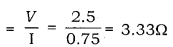# A torch bulb is rated 2.5V and 750mA. Calculate (i) its power

A torch bulb is rated 2.5V and 750mA. Calculate
(i) its power
(ii) its resistance and
(iii) the energy consumed if this bulb is lighted for four hours.

We have,
Voltage (V) = 2.5V
Current (I) = 750mA = 0.75A
Since, bulb is lighted for 4 hours.
Thus,
(i) Power generated § = VI = 2.50 × 0.75 = 1.87W

(ii) Restistance ( R)(iii) Energy consumed in 4 hours = Power × Time = P × t
= 1.87 × 4 = 7.48wh.